## Building COMSOL Multiphysics® Models with Excel® and Visual Basic®

#####Kristoffer Raneflod March 15, 2017

Have you ever thought about customizing or building a model in the COMSOL Multiphysics® software that is based on data from Excel® spreadsheet software? Excel spreadsheets are used to present, collaborate, and store data within many science and engineering applications. Using Microsoft® Visual Basic® for Applications and LiveLink™ for Excel®, we can build and control model settings and parameters within the Excel® spreadsheet software. In this blog post, we illustrate this process and go over a few examples.

### Using LiveLink™ for Excel® with Microsoft® Visual Basic® for Applications

LiveLink™ for Excel® is an interfacing product that enables you to connect your Excel data to COMSOL Multiphysics simulations. If you are a new user of LiveLink™ for Excel®, you can get started by reading the following documentation (accessible after installing the software):

• Introduction to LiveLink™ for Excel®
• LiveLink™ for Excel® User’s Guide

The Excel® spreadsheet software also provides the functionality to define and run VBA (Microsoft® Visual Basic® for Applications) from within an Excel workbook. Although we can write the VBA scripts manually, it is also possible to generate them from an existing model using the COMSOL Multiphysics user interface. As we will see with the help of some examples, it is easy to implement the use of VBA with LiveLink™ for Excel®. We will look into a common application to retrieve and update parameters in a COMSOL Multiphysics model.

Although this functionality is useful, VBA and LiveLink™ for Excel® can be used for a lot more. We will, for example, see how it is possible to build a COMSOL Multiphysics model and define the model geometry with some basic shapes inserted in the Excel workbook.

Note: The examples discussed here are shown with Excel® version 2010, but the process is the same in other versions.

With VBA, it is possible to interface Component Object Model (COM) components. When LiveLink™ for Excel® is installed, it also installs a COM interface component that can be used to interface with COMSOL Multiphysics®. Two essential COM objects for interfacing between the COMSOL Multiphysics Server and COMSOL Multiphysics models are:

```comsolcom.comsolutil
comsolcom.modelutil
```

By using `comsolcom.comsolutil`, it is possible to start a COMSOL Multiphysics Server, connect, and disconnect from the server. With ` comsolcom.modelutil`, we can interface with COMSOL Multiphysics models.

#### Using the VBA Editor

We can write and edit VBA scripts in Excel workbooks with the help of the editor that is installed with the Excel® spreadsheet software. The editor window can be accessed in a couple of different ways. For example, the editor is shown if we right-click an Excel worksheet tab and select View Code. The editor is also displayed if we create or edit a macro. It is also possible to enable a Developer tab in the toolbar in Excel® spreadsheet software that contains buttons for accessing the editor and other development-related functionality.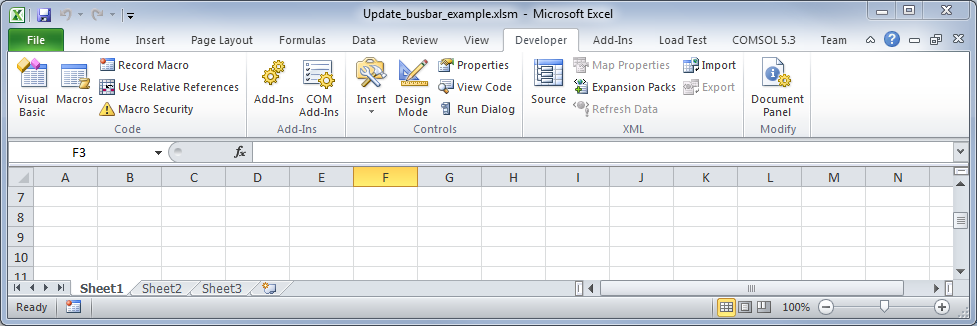#### Accessing COM Components in VBA

We can create dynamic instances of the `comsolcom.comsolutil` and ` comsolcom.modelutil` objects in VBA with the following declaration.

```Set comsolutil = CreateObject("comsolcom.comsolutil")
Set modelutil = CreateObject("comsolcom.modelutil")
```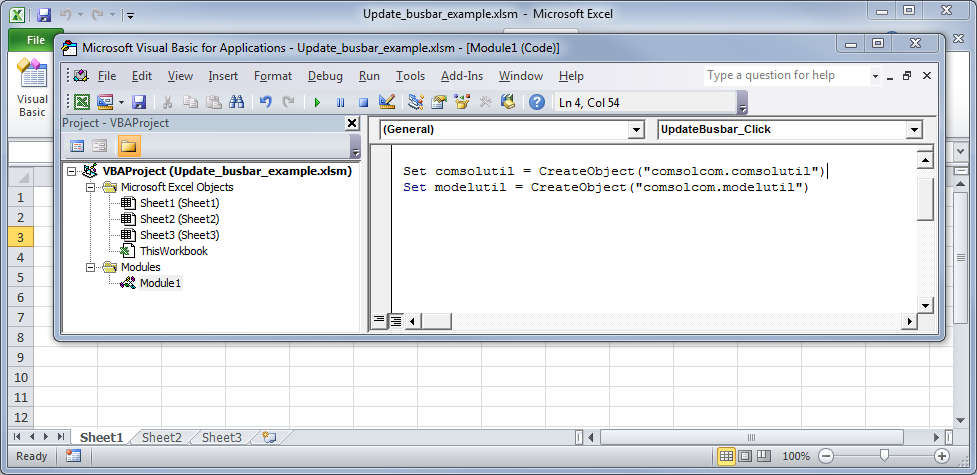The advantage with this declaration is version independence. The latest installed versions of `comsolcom.comsolutil` and ` comsolcom.modelutil` are used at runtime.

It is also possible to declare `comsolcom.comsolutil` and ` comsolcom.modelutil` with a static COM reference using

```Dim comsolutil As comsolutil
Set comsolutil = CreateObject("comsolcom.comsolutil")
Dim modelutil As  modelutil
Set modelutil = CreateObject("comsolcom.modelutil")
```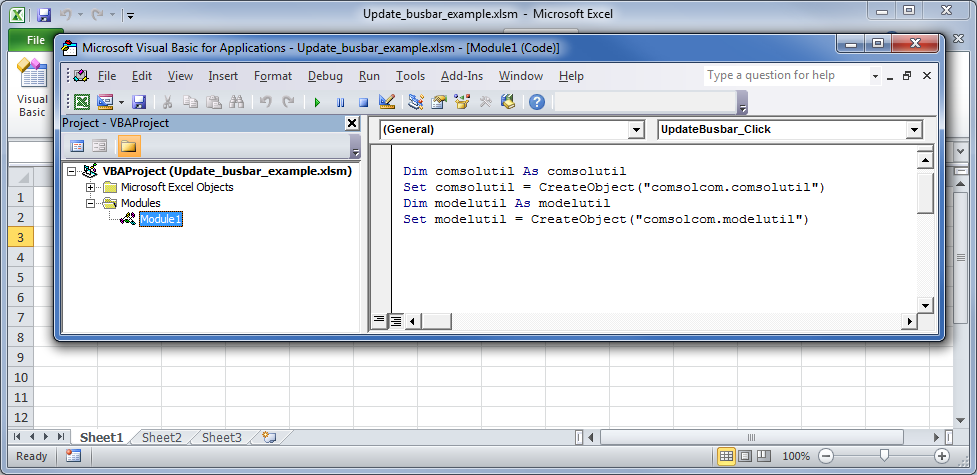An advantage of using this declaration is that help will be available in VBA when using the defined types.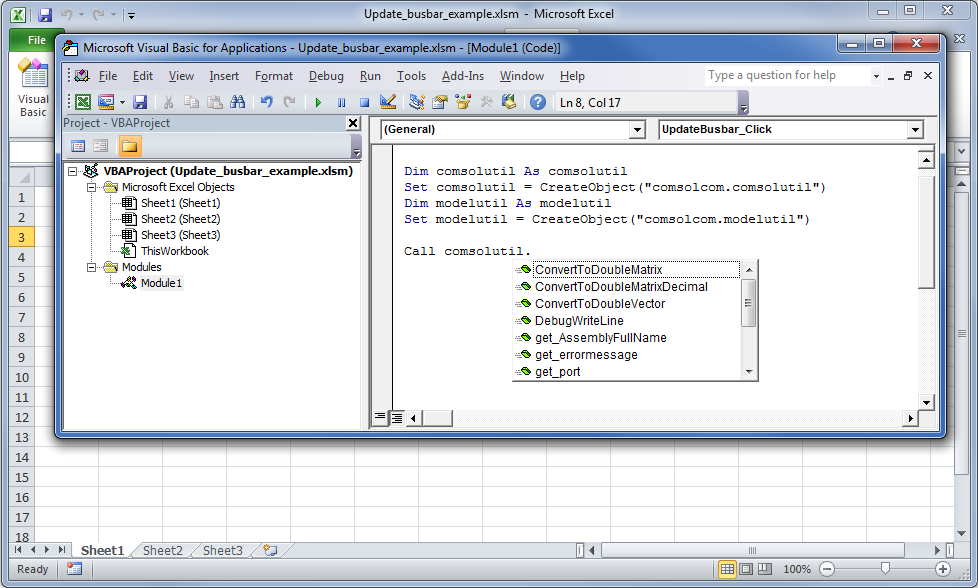In order to be able to define static types for `comsolutil` and `modelutil`, we must add a COM reference to `ComsolCom`. We can do so by opening the VBA editor in the Excel® spreadsheet software, selecting the Tools menu, selecting References, and selecting ` ComsolCom` for the installed version.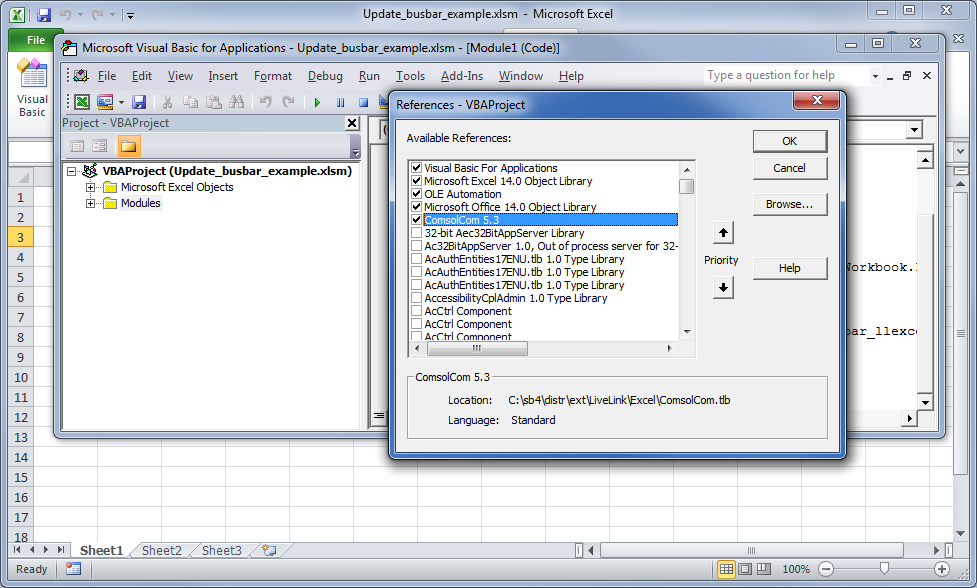### Start a COMSOL Multiphysics Server, Connect, and Disconnect with VBA

The following short VBA script illustrates how to start a COMSOL Multiphysics Server, connect to the started server, and then disconnect from the server. The line call `comsolutil.TimeOutHandle(True)` applies a timeout handler that tells Excel® spreadsheet software to wait for long-running commands to return.

```Set comsolutil = CreateObject("comsolcom.comsolutil")
Set modelutil = CreateObject("comsolcom.modelutil")
Call comsolutil.TimeOuthandler(True)
Call comsolutil.StartComsolServer(True)
Call modelutil.connect
Call modelutil.Disconnect
```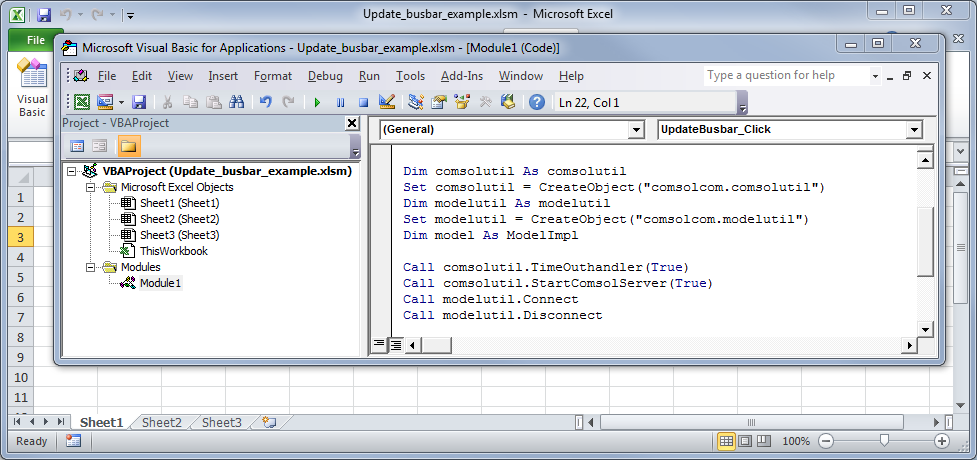#### Migrating from COMSOL API for Use with Java® and Application Methods

If you have experience with the COMSOL API for use with Java® or writing code in application methods, there is a syntax difference that is good to know about. When retrieving a list of model features, for example, the syntax is similar for studies in the model. Thus, for retrieving studies in a model, the following syntax works:

```model.study()
```

However, when accessing a specific study, the syntax is different. For example, when retrieving a study with the study tag `std1` with the COMSOL API for use with Java® or code in applications, the syntax ` model.study("std1")` works. However, with VBA® and LiveLink™ for Excel®, the following syntax must be used instead:

```model.get_study("std1")
```

#### Interface Parameters in COMSOL Multiphysics® Models

A common application of VBA and LiveLink™ for Excel® is to retrieve and update parameters in a COMSOL Multiphysics® model. Here, we will see how easily this can be achieved.

The following VBA script starts a COMSOL Multiphysics Server, connects to the started server, loads the Electrical Heating in a Busbar Using the LiveLink™ for Excel® model from the same directory as the active Excel® workbook, solves the model with an updated length parameter, and saves the updated model with another file name.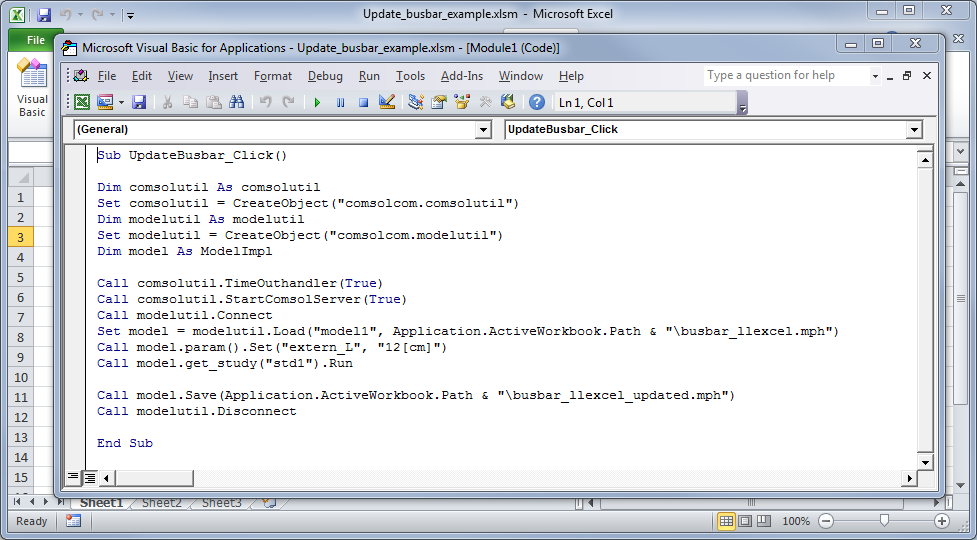The following VBA script extracts parameter data for parameters in the model and inserts them into the Excel workbook.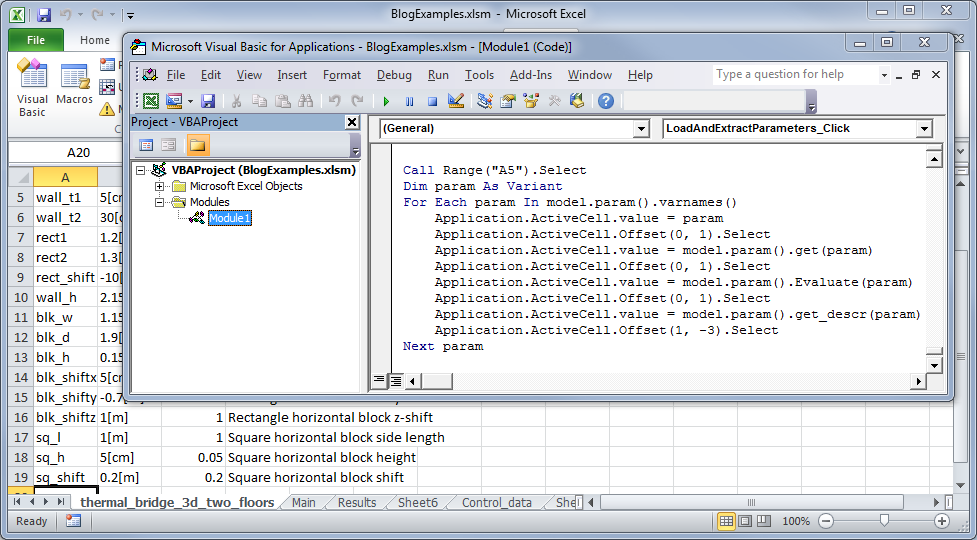### How to Build COMSOL Multiphysics® Models with Excel® and Visual Basic®

In the next example, we create a COMSOL Multiphysics model and solve a 2D simulation using the Heat Transfer in Solids interface. The process involves defining geometry within Excel® spreadsheet software by adding a text box with some instructions, an outer temperature boundary, an inner temperature boundary, and a button for solving the simulation. When the model is solved, a results plot is inserted in the Excel® workbook. Let’s go through these steps in detail.

1. First, we create a text box with instructional text and insert it into the Excel workbook.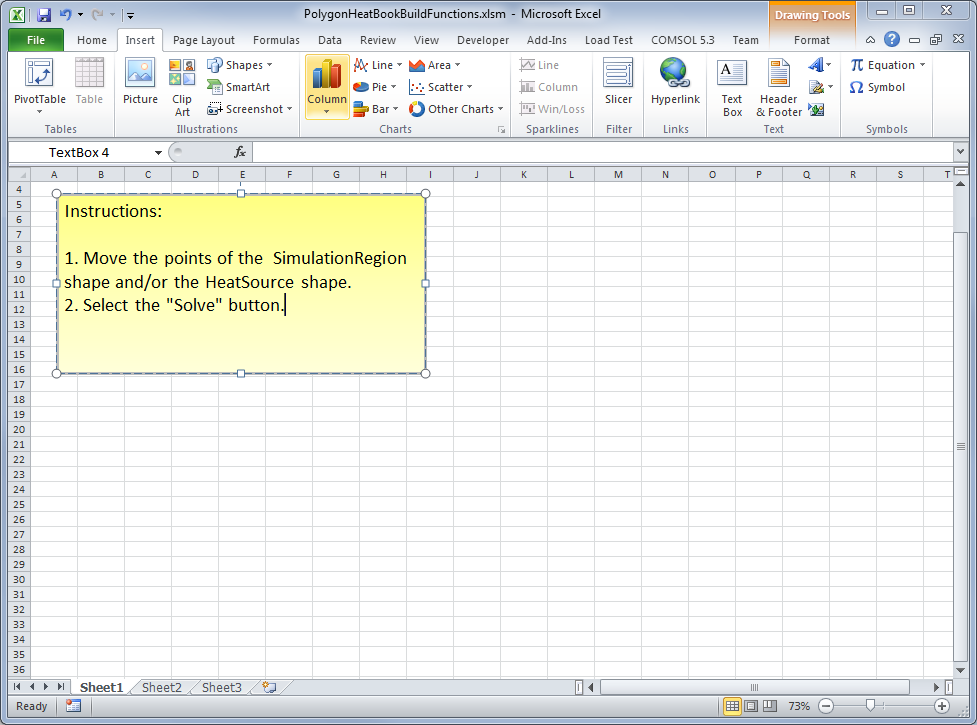2. Then, we define a region for the simulation. We select a freeform shape and insert it in the Excel® workbook. Then, we select SimulationRegion as the name for the shape. We make the polygon editable by right-clicking on the shape and selecting Edit Polygon. Then, we edit the shape as shown below.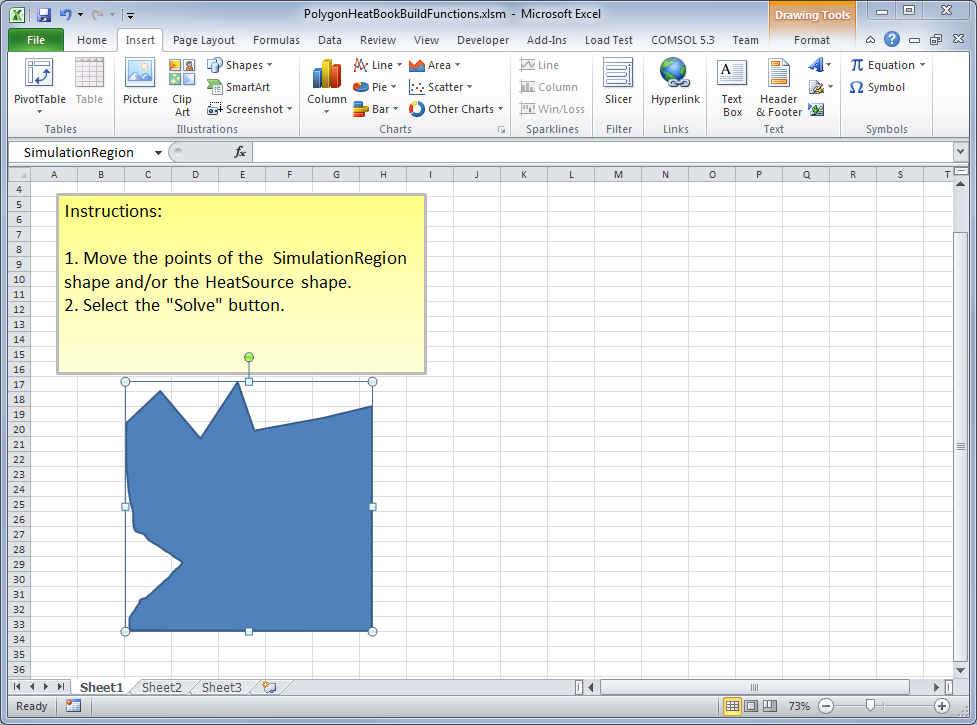3. We create an inner boundary with a higher temperature. To do so, we use an oval shape, create a circle, and insert it inside the freeform. We select HeatSource as the name for the shape. The oval shape must reside inside the SimulationRegion shape.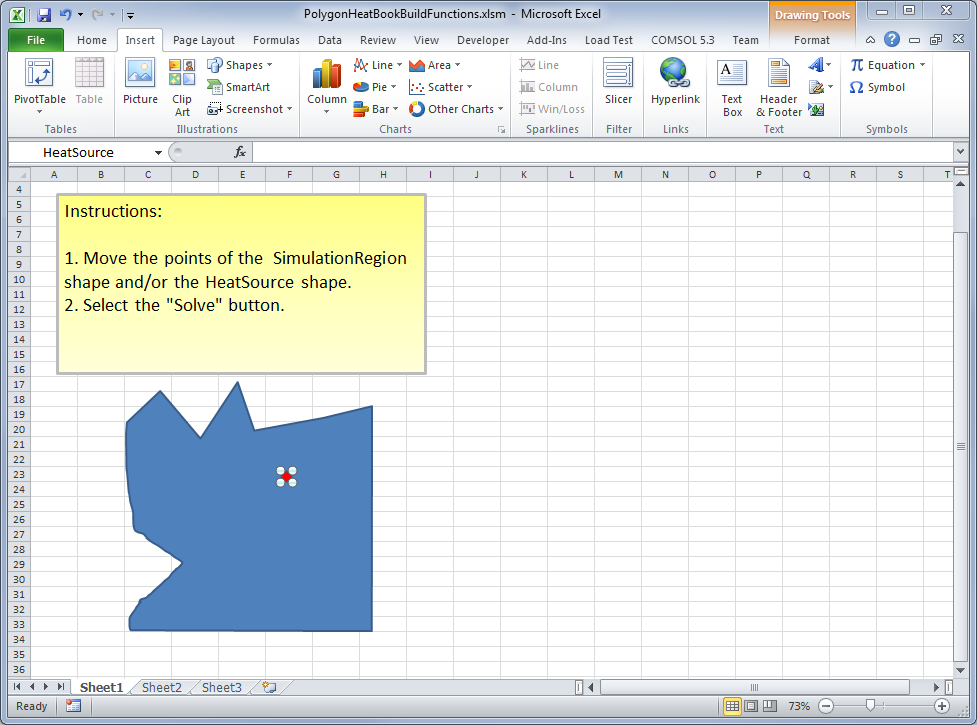4. We then add a text box shape with the text Solve to use as a button. We right-click on the button, select Assign Macro, and create a new macro named Solve_Click.

5. Next, we open the assigned macro in the VBA® editor and replace the content with the following script:

Expand or collapse VBA code…

```Option Explicit

Sub Solve_Click()

Dim node
Dim coordinates
Dim index
Dim newPolygonTable() As Double
Dim newHeatSource(1 To 2) As Double
Dim model As ModelImpl

newHeatSource(1) = Sheets("Sheet1").Shapes("HeatSource").DrawingObject.Left + (Sheets("Sheet1").Shapes("HeatSource").DrawingObject.Width / 2)
newHeatSource(2) = Sheets("Sheet1").Application.Height - (Sheets("Sheet1").Shapes("HeatSource").DrawingObject.Top + (Sheets("Sheet1").Shapes("HeatSource").DrawingObject.Height / 2))

Dim nNodes As Long
nNodes = Sheets("Sheet1").Shapes("SimulationRegion").Nodes.Count
ReDim Preserve newPolygonTable(1 To nNodes, 1 To 2)

For Each node In Sheets("Sheet1").Shapes("SimulationRegion").Nodes
coordinates = node.points
index = index + 1
newPolygonTable(index, 1) = coordinates(1, 1)
newPolygonTable(index, 2) = Sheets("Sheet1").Application.Height - coordinates(1, 2)
Next

Set model = SetModel(newPolygonTable, newHeatSource)

Call model.get_study("std1").Run

If Not ContainsTag(model.result().tags(), "pg1") Then
Call model.result().Create("pg1", "PlotGroup2D")
Call model.get_result("pg1").feature().Create("surf1", "Surface")
Call model.get_result("pg1").Label("Temperature (ht)")
Call model.get_result("pg1").set("data", "dset1")
Call model.get_result("pg1").get_feature("surf1").Label("Surface")
Call model.get_result("pg1").get_feature("surf1").set("colortable", "ThermalLight")
Call model.get_result("pg1").get_feature("surf1").set("data", "parent")
Call model.get_result("pg1").get_feature("surf1").Run
End If
Call model.get_result("pg1").Run

Call Range("J10").Select
Dim tempPng As String
tempPng = Environ("Temp") & "\PolygonHeat" & Format(Now(), "yyyymmddhhmmss") & ".png"

Dim exportTag As String
exportTag = model.result().Export.uniquetag("export")
Call model.result().Export().Create(exportTag, "Image2D")
Call model.result().get_export(exportTag).set("plotgroup", "pg1")
Call model.result().get_export(exportTag).set("pngfilename", tempPng)
Call model.result().get_export(exportTag).Run

If Dir(tempPng)  "" Then
Call Application.ActiveSheet.Pictures.Insert(tempPng)
SetAttr tempPng, vbNormal
Kill tempPng
End If

Call model.result().Export().Remove(exportTag)

End Sub

Private Function SetModel(ByRef newPolygonTable() As Double, ByRef newHeatSource() As Double) As Variant

Dim comsolutil As comsolutil
Set comsolutil = CreateObject("comsolcom.comsolutil")
Dim modelutil As modelutil
Set modelutil = CreateObject("comsolcom.modelutil")
Dim model As ModelImpl

If Not IsConnected(modelutil) Then
Call ConnectServer(comsolutil, modelutil)
End If

If Not ContainsTag(modelutil.tags(), "PolygonHeatModel") Then
Set SetModel = CreateModel(modelutil, "PolygonHeatModel", newPolygonTable, newHeatSource)
Exit Function
End If

Set model = modelutil.model("PolygonHeatModel")
Call model.get_geom("geom1").get_feature("pol1").set("table", newPolygonTable)

Call model.get_geom("geom1").get_feature("c1").set("x", newHeatSource(1))
Call model.get_geom("geom1").get_feature("c1").set("y", newHeatSource(2))
Call model.get_geom("geom1").runAll

Set SetModel = model

End Function

Private Function CreateModel(ByRef modelutil As modelutil, ByRef modelTag As String, ByRef newPolygonTable() As Double, ByRef newHeatSource() As Double) As Variant
Dim model As ModelImpl
Set model = modelutil.Create(modelTag)

Call model.ModelNode().Create("comp1")

Call model.geom().Create("geom1", 2)
Call model.mesh().Create("mesh1", "geom1")

Call model.get_geom("geom1").Create("pol1", "Polygon")
Call model.get_geom("geom1").get_feature("pol1").set("source", "table")
Call model.get_geom("geom1").get_feature("pol1").set("table", newPolygonTable)
Call model.get_geom("geom1").Selection().Create("csel1", "CumulativeSelection")
Call model.get_geom("geom1").get_feature("pol1").set("contributeto", "csel1")
Call model.get_geom("geom1").get_run("pol1")

Call model.get_geom("geom1").Create("c1", "Circle")
Call model.get_geom("geom1").get_feature("c1").set("r", 0.01)
Call model.get_geom("geom1").get_feature("c1").set("x", newHeatSource(1))
Call model.get_geom("geom1").get_feature("c1").set("y", newHeatSource(2))
Call model.get_geom("geom1").Selection().Create("csel2", "CumulativeSelection")
Call model.get_geom("geom1").get_feature("c1").set("contributeto", "csel2")

Call model.get_geom("geom1").Run
Call model.get_geom("geom1").get_run("fin")

Call model.Material().Create("mat1", "Common", "comp1")
Call model.get_material("mat1").set("family", "copper")
Call model.get_material("mat1").get_propertyGroup("def").set("heatcapacity", "385[J/(kg*K)]")
Call model.get_material("mat1").get_propertyGroup("def").set("density", "8960[kg/m^3]")
Call model.get_material("mat1").get_propertyGroup("def").set("thermalconductivity", "400[W/(m*K)]")

Call model.Physics().Create("ht", "HeatTransfer", "geom1")
Call model.get_physics("ht").Create("temp1", "TemperatureBoundary", 1)
Call model.get_physics("ht").Create("temp2", "TemperatureBoundary", 1)
Call model.get_physics("ht").get_feature("temp2").set("T0", "293.15[K]+20")
Call model.get_physics("ht").get_feature("temp1").Selection().named("geom1_csel1_bnd")
Call model.get_physics("ht").get_feature("temp2").Selection().named("geom1_csel2_bnd")

Call model.study().Create("std1")
Call model.get_study("std1").Create("stat", "Stationary")
Call model.get_study("std1").Run

Call model.result().Create("pg1", "PlotGroup2D")
Call model.get_result("pg1").Label("Temperature (ht)")
Call model.get_result("pg1").set("data", "dset1")
Call model.get_result("pg1").feature().Create("surf1", "Surface")
Call model.get_result("pg1").get_feature("surf1").Label("Surface")
Call model.get_result("pg1").get_feature("surf1").set("colortable", "ThermalLight")
Call model.get_result("pg1").get_feature("surf1").set("data", "parent")

Set CreateModel = model

End Function

Private Function IsConnected(modelutil As modelutil) As Boolean

'Try to access model tags. If not connected to a server this will throw an error.
On Error GoTo ErrorHandler
Call modelutil.tags
IsConnected = True
Exit Function

ErrorHandler:
IsConnected = False

End Function

Private Function ConnectServer(comsolutil As comsolutil, modelutil As modelutil)

On Error GoTo ErrorHandler
Call modelutil.connect
If Not comsolutil.isGraphicsServer() Then
MsgBox prompt:="The running COMSOL Multiphysics Server is not a graphics server. Exporting results will not work.", Buttons:=vbOKOnly, Title:="COMSOL"
End If
Exit Function

ErrorHandler:

Call comsolutil.TimeOuthandler(True)
Call comsolutil.StartComsolServer(True)
Call modelutil.connect

End Function

Private Function ContainsTag(tags() As String, tag As String) As Boolean

ContainsTag = False
If (UBound(Filter(tags, tag)) > -1) Then
ContainsTag = True
End If

End Function
```

6. After inputting the code, we click on the Solve button. This executes the VBA script defined in the macro and creates the model based on the shapes in the Excel workbook. The model is solved and the graphics are inserted into the worksheet.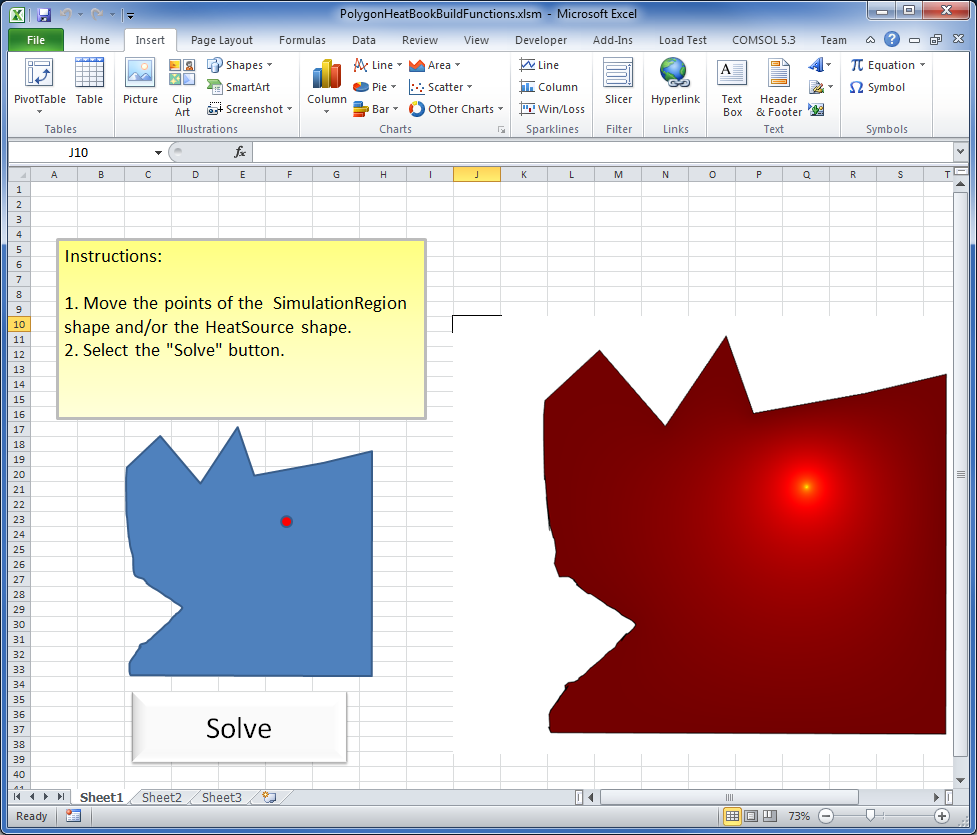If the SimulationRegion shape is changed and the HeatSource shape is moved to another location inside the SimulationRegion, the model and results will be different.

It is easy to imagine how we can control and program this model based on other shapes, charts, and data in an Excel workbook. It is also possible to extract numerical results from the COMSOL Multiphysics model and generate Excel workbook content such as for reporting purposes, for example.

This blog post just scratches the surface with regard to what you can do using VBA and Excel® spreadsheet software. As a user, you have access to the entire COMSOL API, which gives access to all model settings and parameters. This makes it possible for you to define any type of model and extracts its data after having solved it using COMSOL Multiphysics.

Microsoft, Excel, and Visual Basic are either registered trademarks or trademarks of Microsoft Corporation in the United States and/or other countries.

Oracle and Java are registered trademarks of Oracle and/or its affiliates.

#### Post Tags

Technical Content